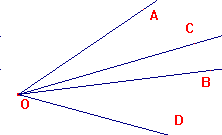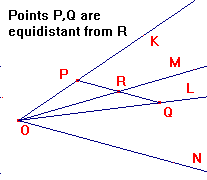harmonic pencils

 If 4 collinear p-points embed as non-ideal points on a plane Π, then the cross-ratio is a ratio of signed ratios in Π (see the embedding theorem) The Greeks studied this, thinking of the quantity as defined by a pencil of four lines i.e. a set of four coplanar and concurrent lines. In projective geometry, we regard the lines as representing collinear p-points, and the common point as the origin in the model RP2. A particularly interesting case is when the cross-ratio is -1, when we say that the pencil is harmonic. The image on the right shows such a pencil. To show how information from a projective figure can be used to obtain euclidean results, we begin with the following theorem. A mid-point theorem Suppose that A,B,C,D are p-points with (A,B,C,D) = -1, and that a plane Π is chosen so that the p-points embed as A',B',C',D' with (only) D' ideal. Then C' is the mid-point of A'B'.Proof From case (5) of the the embedding theorem, we have (A,B,C,D) = C'A'/C'B'. Thus C'A'/C'B' = -1, so C' is the mid-point of A'B'. We can rephrase this as a euclidean result: If (K,L,M,N) is a harmonic pencil and P,Q lie on K,L such that PQ||N, then M bisects PQ. To see how such a cross-ratio may arise in practice, we will look at some results on projective conics.